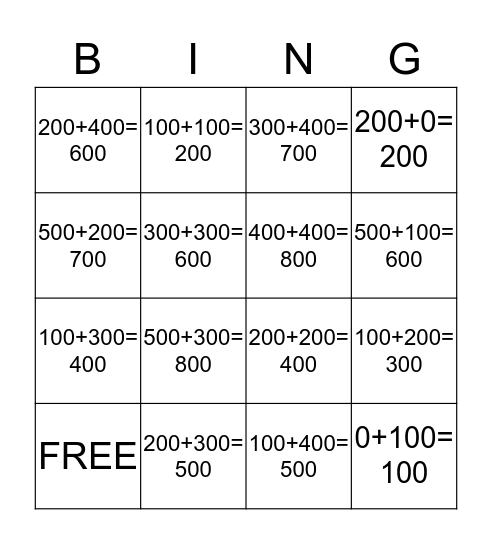# EstimationThis bingo card has 16 words: 200+300= 500, 100+200= 300, 300+400= 700, 200+400= 600, 100+300= 400, 100+100= 200, 200+200= 400, 100+400= 500, 500+200= 700, 300+300= 600, FREE, 200+0= 200, 500+300= 800, 500+100= 600, 400+400= 800 and 0+100= 100.

## Play Online